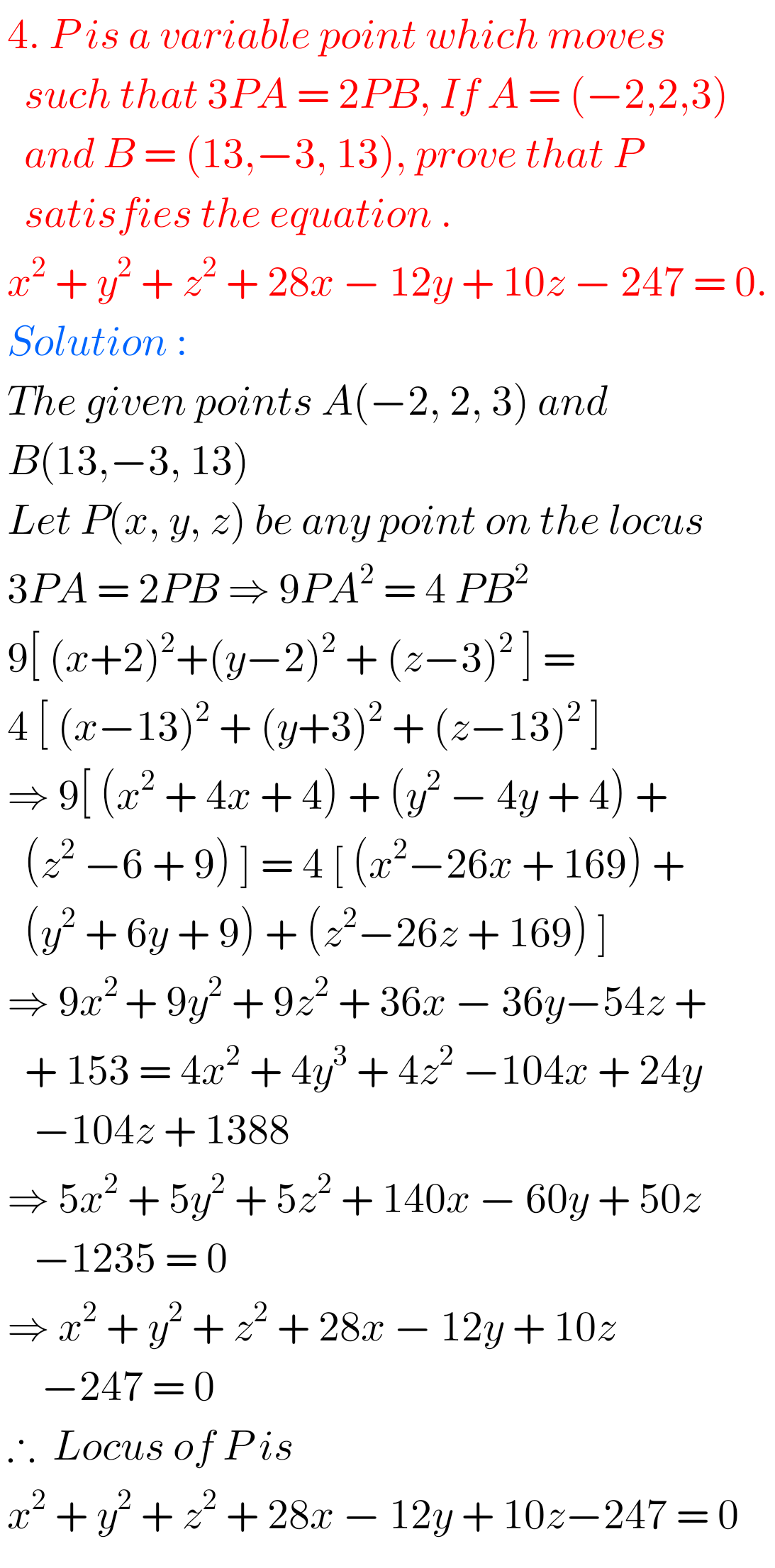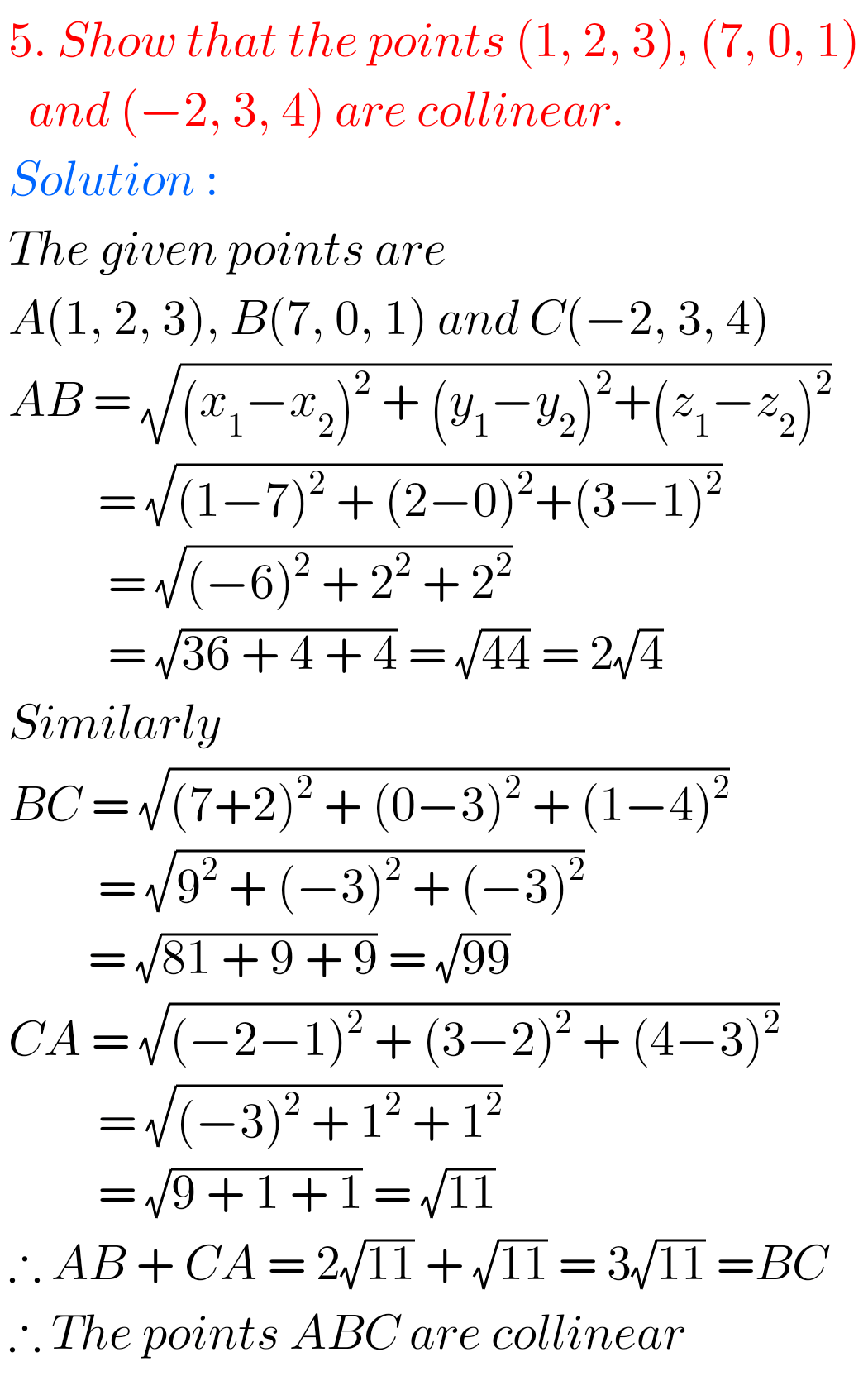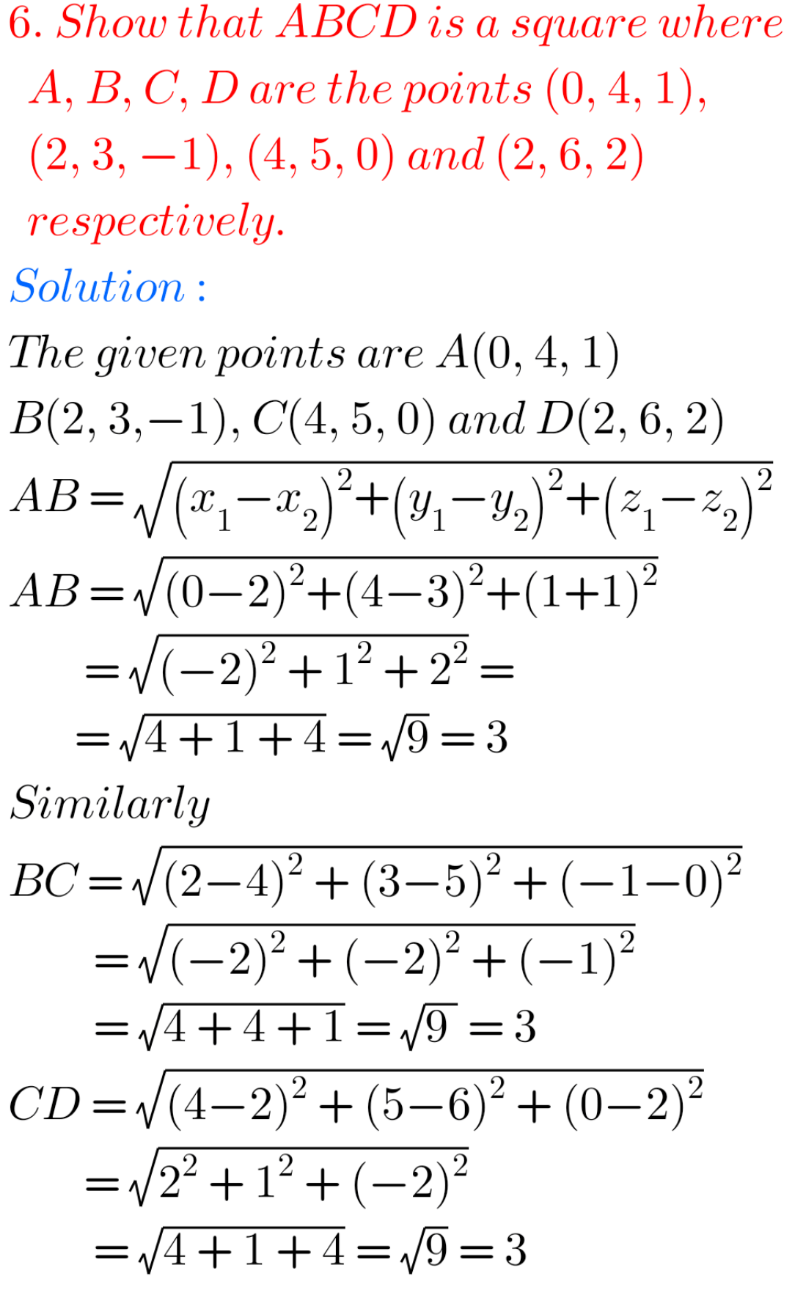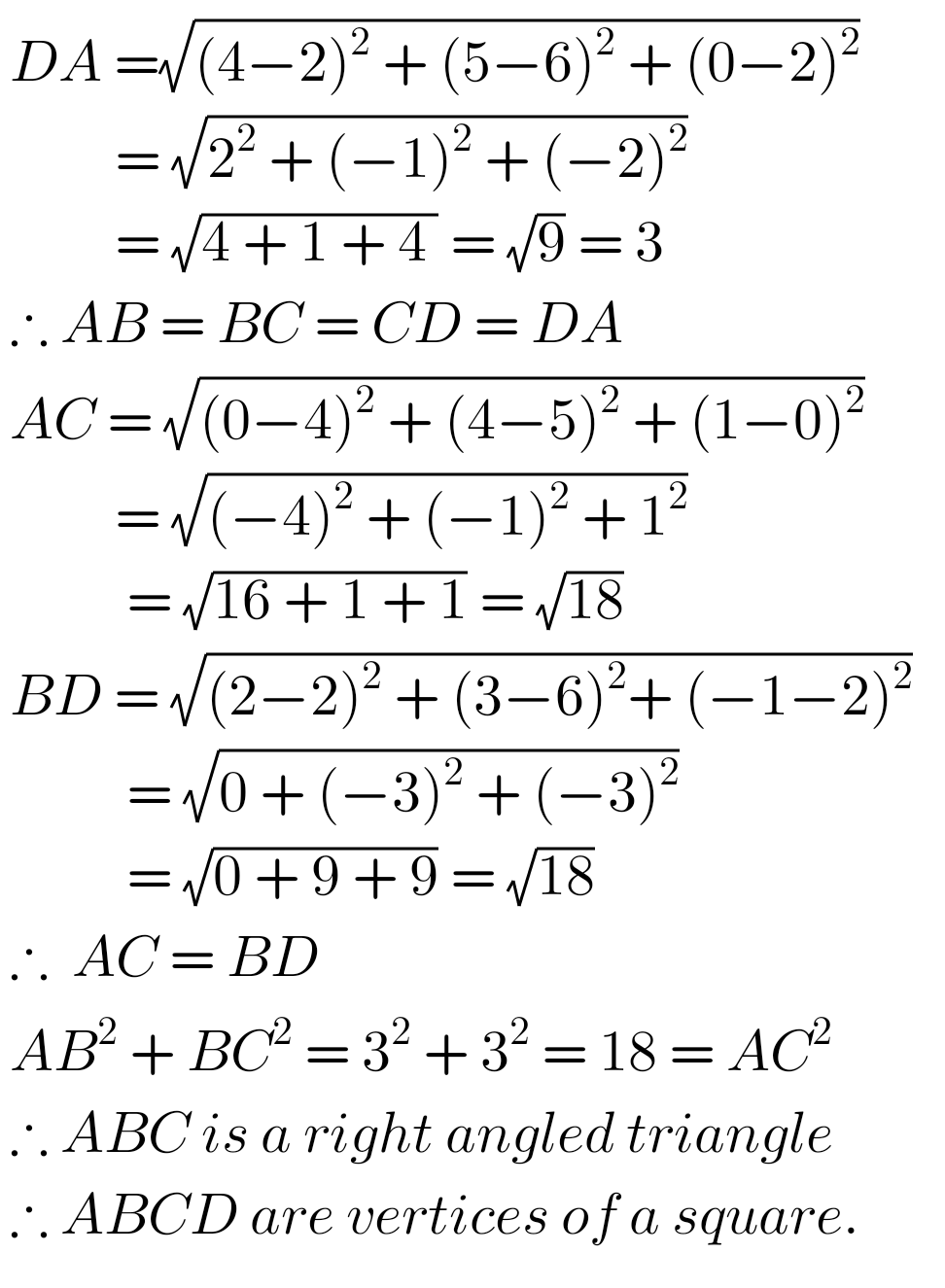# Three Dimensional Coordinates solutions Inter

Intermeduate Mathematics 1B Chapter 5 Three Dimensional Coordinates Exercise 5(a) textbook solutions are given.

First you sytudy the textbook lesson very well. Then observe the example problems and solutions given in the textbook.

The keypoints in the lesson are very important.

Observe the solutions given below and try them in your own method.

You can also see

Inter Maths 1A textbook solutions

Inter Maths 1B textbook solutions

Inter Maths IIA textbook solutions

Inter Maths IIB textbook solutions

Three Dimensional Coordinates

Exercise 5(a)

Exercise 5(b)

M

# Maths Solutions for Three Dimensional Coordinates Exercise 5(a)## Exercise 5(a) Chapter 5 Three Dimensional Coordinates Inter## Intermediate Solutions for Maths 1b Three Dimensional Coordinates exercise 5(a)M

Note : Observe the solutions and try them in your own method.

SSC Maths textbook solutions class 10

Ncert maths class 6 chapter 14 solutions

Nios maths 311 book 1 Sets 1.1 solutions